# Representing Sets. In Venn Diagrams.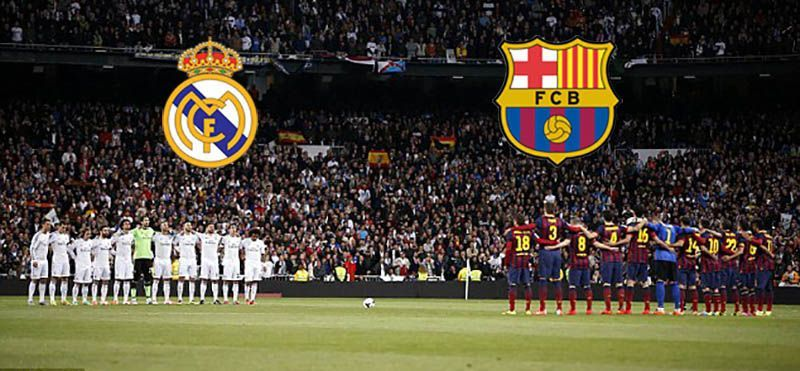A set can be represented diagrammatically using a circle or an ellipse.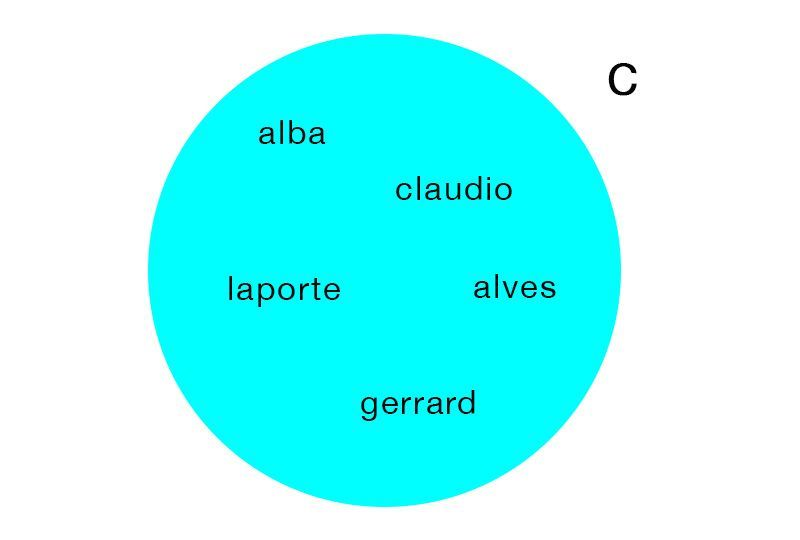The elements are written inside the circle / ellipse.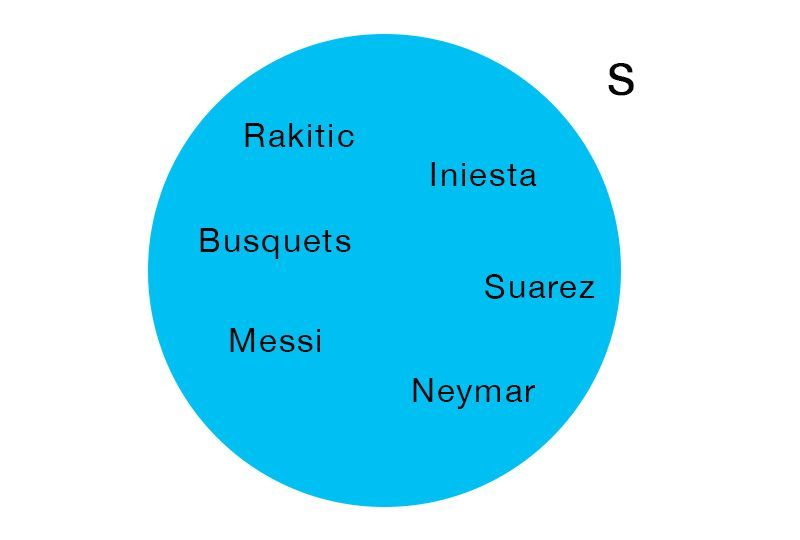The name of the set is denoted by a capital letter written outside the circle, near to the circle.

# Venn Diagrams of 2 sets

Disjoint sets are represented by two separate circles.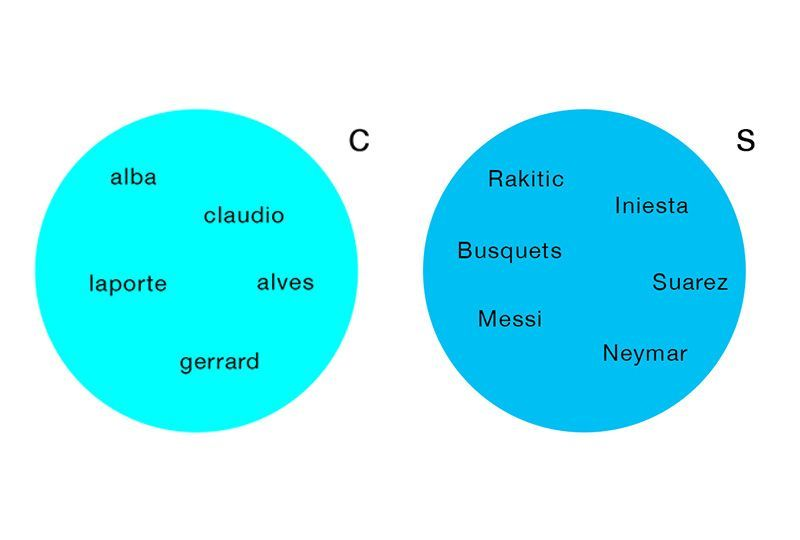Draw a Rectangle, that encloses the set.

Draw a Circle/Ellipse for each of the set with elements in it.

# Access All Content

## More from Grade 4 Math# Pictograph

Representing your world in pictures! See how the type and number of a quantity can be mathematically represented pictorially.# Venn Diagram

The easiest way to imagine sets visually. See the power of Venn Diagrams.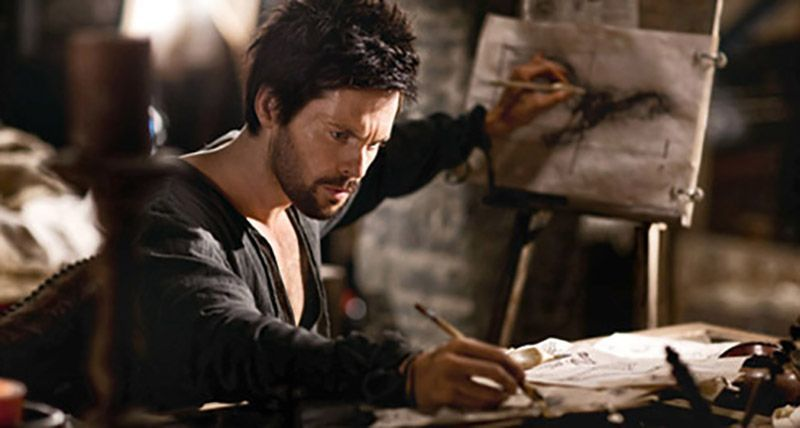# Venn Diagram Example

Learn how to use Venn Diagrams to solve real world problems with these interesting examples.